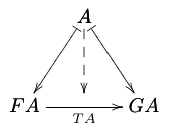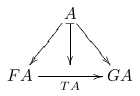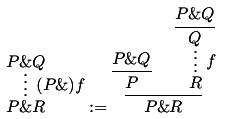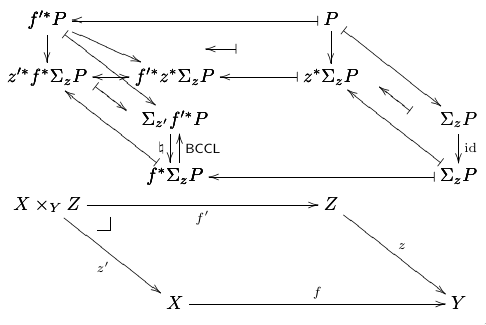### Dednat6: an extensible (semi-)preprocessor for LuaLaTeX that understands diagrams in ASCII art

(Lua)(La)TeX treats lines starting with "%" as comments, and ignores them. This means that we can put anything we want in these "%" lines - even code to be processed by other programs besides *TeX. Dednat6 is a Lua program that treats blocks of comments in a .tex file starting with "%D" as specifications for diagrams, and blocks of comments starting with "%:" as specifications for derivation trees.

Here is an example of a .tex file with a block of "%D" lines:

 \documentclass{article} \usepackage{proof} \input diagxy \xyoption{curve} \begin{document} \catcode\^^J=10 \directlua{dofile "dednat6load.lua"} %D diagram T:F->G %D 2Dx 100 +20 +20 %D 2D 100 A %D 2D /|\ %D 2D v v v %D 2D +30 FA --> GA %D 2D %D (( A FA |-> A GA |-> %D FA GA -> .plabel= b TA %D A FA GA midpoint --> %D )) %D enddiagram %D $$\pu \diag{T:F->G}$$ \end{document} 

If we compile that file with lualatex - a version of TeX that can run Lua programs from inside TeX - we get a page with this diagram:I wrote the ancestors of dednat6 zillions of years ago, to help me typeset categorical diagrams and Natural Deduction trees for my mathematical research, but until 2018 these dednats were very very very hard to use if you weren't me. In 2018 I gave a presentation about dednat6 at TUG2018 (slides), published an article at TUGBoat about it, and simplified Dednat6's initialization code a lot; in 2019 I (finally) wrote decent minimal test files (also this and this), cleaned up the package and this web page, and wrote the quick start guide below.

Quick index:

## 1. A quick start guide for beginners

If you use TeXstudio, TeXworks or something similar, then start by this.

2. Edit demo-minimal.tex
3. Set your editor font to some monospaced font
4. Set your compiler to lualatex
5. Compile demo-minimal.tex and view the PDF
6. Make small changes to the deduction tree in ascii art, recompile, view

## 2. The git repository

Since 2019may21 dednat6 has a git repository.
If you're in a *NIX-based system you can download dednat6 from there and test it with:

 rm -Rfv /tmp/dednat6/ cd /tmp/ git clone https://github.com/edrx/dednat6 cd /tmp/dednat6/ make make clean 

The "make" will compile all its .tex files into PDFs; the "make clean" will delete all the generated files except the PDFs. After doing "make" and "make clean" open the file demo-minimal.tex, read its instructions and try to modify it and recompile it.

The git repository has most files from http://angg.twu.net/dednat6/, but not all. Use the table below to access the HTMLized versions of the source files - in which most elisp hyperlinks become HTML hyperlinks - and the PDFs produced from the .tex files.

 VERSION README.md Makefile (HTML) dednat6load.lua (HTML) dednat6/dednat6.lua (HTML) dednat6/lualoader.lua (HTML) dednat6/binloader.lua (HTML) dednat6/edrxlib.lua (HTML) dednat6/abbrevs.lua (HTML) dednat6/block.lua (HTML) dednat6/diagforth.lua (HTML) dednat6/diagmiddle.lua (HTML) dednat6/diagstacks.lua (HTML) dednat6/diagtex.lua (HTML) dednat6/eoo.lua (HTML) dednat6/errors.lua (HTML) dednat6/heads6.lua (HTML) dednat6/luarects.lua (HTML) dednat6/options6.lua (HTML) dednat6/output.lua (HTML) dednat6/parse.lua (HTML) dednat6/preamble6.lua (HTML) dednat6/rect.lua (HTML) dednat6/stacks.lua (HTML) dednat6/texfile.lua (HTML) dednat6/treesegs.lua (HTML) dednat6/treetex.lua (HTML) dednat6/picture.lua (HTML) dednat6/tcgs.lua (HTML) dednat6/underbrace.lua (HTML) dednat6/underbrace2d.lua (HTML) dednat6/zhas.lua (HTML) dednat6/zhaspecs.lua (HTML) dednat6/zquotients.lua (HTML) dednat6/luarepl.lua (HTML) dednat6/lua-repl/repl/compat.lua dednat6/lua-repl/repl/init.lua dednat6/lua-repl/repl/sync.lua dednat6/lua-repl/repl/utils.lua demo-minimal.tex (HTML) (PDF) demo-write-dnt.tex (HTML) (PDF) demo-underbrace.tex (HTML) (PDF) demo-preproc.tex (HTML) (PDF) demo-repl.tex (HTML) tugboat-rev2.tex (HTML) (PDF) extra-comparisons.tex (HTML) (PDF) extra-features.tex (HTML) (PDF) extra-modules.tex (HTML) (PDF) 2018dednat6-extras.tex (HTML) (PDF) tug-slides.tex (HTML) (PDF) myverbatim.lua (HTML) tug-slides-ss1.png tug-slides-sslr2.png 

## 3. dednat6.zip

Most people will find it easier to work with the .zip package of dednat6 than with the git repository. The .zip contains all the files in the git archive plus the PDFs. Its URL is:

 http://angg.twu.net/dednat6.zip 

The beginner-ish way to use it is to unpack it, read demo-minimal.tex and start modifying it - as in the quick start guide. If you are on a *NIX-like system and want to test it in the same way as we did with the git repository in the previous sections, the commands are:

 rm -Rfv /tmp/dednat6/ mkdir /tmp/dednat6/ cd /tmp/dednat6/ wget http://angg.twu.net/dednat6.zip unzip dednat6.zip make veryclean make make clean 

The "make veryclean" is like "make clean" but it also deletes the PDFs. "make" remakes the PDFs, but leaves some intermediate files; "make clean" deletes these intermediate files but leaves the PDFs... so the sequence of three "make"s above is a way to check that are the PDF can be re-generated without errors.

## 4. Hacking

If you know a bit of Emacs and eev then you can try to use the "test blocks" embedded in the source files of eev to inspect the data structures... see:

 (find-eev-quick-intro "6. Controlling shell-like programs") (find-eepitch-intro "3. Test blocks") (find-dednat6 "dednat6/diagtex.lua" "arrow_to_TeX-test") (find-dednat6 "dednat6/diagtex.lua" "arrows-tests") (find-dednat6 "dednat6/zhas.lua" "ZHA-tests") 

By the way: if you know how to create categorical diagrams with Tikz and would like to help me to create a Tikz back-end for the 2D diagrams module, please get in touch!!! I will do 95%+ of the work - it's basically a matter of creating a variant of arrow_to_TeX that will coexist with the current one - but I need to have someone who will help me with some Tikz questions...

By the way 2: the directory dednat6/ currently contains several modules that are I wrote as quick hacks to typeset diagrams that I needed, and that I don't think that will be interesting to other people - except as curiosities, to show how extensible Dednat6 is. My paper Planar Heyting Algebras for Children (PDF, source tgz) uses many of these extensions; its draft sequel about closure operators (PDF, source tgz) uses a few more.

## 5. The essential files

The demo below shows a way to compile demo-minimal.tex by unpacking only the "essential files" from the .zip file - namely, demo-minimal.tex itself, dednat6load.lua, and all the .lua files in the directory dednat6/.

 rm -Rfv /tmp/dednat6.zip cd /tmp/ wget http://angg.twu.net/dednat6.zip rm -Rfv /tmp/dn6-test-min/ mkdir /tmp/dn6-test-min/ cd /tmp/dn6-test-min/ unzip ../dednat6.zip "dednat6/**" dednat6load.lua demo-minimal.tex ls -l lualatex demo-minimal.tex ls -l 

(Lua)(La)TeX treats lines starting with "%" as comments, and ignores them. This means that we can put anything we want in these "%" lines - even code to be processed by other programs besides *TeX.

Dednat6 read TeX files and pay attention only to the lines that begin with some special sequences of characters (called "heads"), all starting with "%". The main heads are:

%L Lua code
%: derivation trees (two-dimensional)
%D definitions of diagrams (in a stack language)

Dednat4 processes a TeX file, say, foo-4.tex, and produces an auxiliary TeX file, foo-4.dnt, containing the TeX code to typeset the derivation trees and diagrams of foo.tex. Dednat6 does something similar, but the TeX code is usually not saved to a file; instead, it is processed by TeX immediately. Let's look at two examples (in Dednat6 syntax):

User code
LaTeX (generated)
Result
 %D diagram T:F->G %D 2Dx 100 +20 +20 %D 2D 100 A %D 2D / - \ %D 2D / | \ %D 2D v v v %D 2D +25 FA ------> GA %D 2D TA %D (( A FA -> A GA -> %D FA GA -> .plabel= b TA %D A FA GA midpoint |-> %D )) %D enddiagram $$\pu \diag{T:F->G}$$ 
 $$\defdiag{T:F->G}{ \morphism(300,0)/->/% <-300,-375>[{A}{FA};{}] \morphism(300,0)/->/% <300,-375>[{A}{GA};{}] \morphism(0,-375)|b|/->/% <600,0>[{FA}{GA};{TA}] \morphism(300,0)/|->/% <0,-375>[{A}{\phantom{O}};{}] } \diag{T:F->G}$$%: P\&Q %: ---- %: P\&Q Q %: ---- :f %: P\&Q P R %: :(P\&)f ------- %: P\&R P\&R %: %: ^t1 ^t2 %: $$\pu \ded{t1} := \ded{t2}$$ 
 $$\defded{t1}{ \infer*[{(P\&)f}]{ \mathstrut P\&R }{ \mathstrut P\&Q } } \defded{t2}{ \infer[{}]{ \mathstrut P\&R }{ \infer[{}]{ \mathstrut P }{ \mathstrut P\&Q } & \infer*[{f}]{ \mathstrut R }{ \infer[{}]{ \mathstrut Q }{ \mathstrut P\&Q } } } } \ded{t1} := \ded{t2}$$## 7. A big example

Here is an example of a big diagram that I created with dednat6.
It appears at page 7 of my slides for TUG2018 (and in page 20 here).
(The source for the slides in included in dednat6.zip).%D diagram BCCL-std %D 2Dx 100 +45 +55 +45 %D 2D 100 B0 <====================== B1 %D 2D -\\ -\\ %D 2D | \\ | \\ %D 2D v \\ v \\ %D 2D +20 B2 <\\> B2' ============== B3 \\ %D 2D /\ \/ /\ \/ %D 2D +15 \\ B4 \\ B5 %D 2D \\ - \\ - %D 2D \\ | \\| %D 2D \\v \v %D 2D +20 B6 <===================== B7 %D 2D %D 2D +10 b0 |---------------------> b1 %D 2D |-> |-> %D 2D +35 b2 |--------------------> b3 %D 2D %D (( %D B0 .tex= f^{\prime*}P B1 .tex= P %D B2 .tex= z^{\prime*}f^*Σ_zP B3 .tex= z^*Σ_zP %D B4 .tex= Σ_{z'}f^{\prime*}P B5 .tex= Σ_zP %D B6 .tex= f^*Σ_zP B7 .tex= Σ_zP %D B2' .tex= f^{\prime*}z^*Σ_zP %D B0 B1 <-| B0 B2 -> B0 B2' -> B1 B3 -> B2 B2' <-> B2' B3 <-| %D B0 B4 |-> B1 B5 |-> %D B2 B6 <-| B3 B7 <-| %D B6 B7 <-| B5 B7 -> .plabel= r \id %D B4 B6 -> sl_ .plabel= l ♮ B4 B6 <- sl^ .plabel= r \BCCL %D B0 B2' midpoint B1 B3 midpoint harrownodes nil 20 nil <-| %D B0 B2 midpoint B4 B6 midpoint dharrownodes nil 20 nil |-> %D B1 B3 midpoint B5 B7 midpoint dharrownodes nil 20 nil <-| %D )) %D (( b0 .tex= X×_{Y}Z b1 .tex= Z b2 .tex= X b3 .tex= Y %D b0 b1 -> .plabel= b f' %D b0 b2 -> .plabel= l z' %D b1 b3 -> .plabel= r z %D b2 b3 -> .plabel= a f %D b0 relplace 20 7 \pbsymbol{7} %D )) %D enddiagram 

It uses some undocument hacks:

 (find-dn6 "diagmiddle.lua" "harrownodes") (find-dn6 "diagmiddle.lua" "dharrownodes") (find-dn6 "diagforth.lua" "relplace") 

## 8. Dednat4 vs. Dednat6

Note: Dednat4 and Dednat6 are very similar. Dednat4 is easier to explain, because it is just a preprocessor that we have to run like this:

 dednat4 foo-4.tex latex foo-4.tex 

Dednat6, in contrast, is easier to use - we just need this:

 lualatex foo-6.tex 

In Dednat4 all the Lua code is run before running LaTeX; in Dednat6, Lua is run from LuaLaTeX (with the command "\pu") to process chunks of foo-6.tex bit by bit. This is explained in the TUGBoat article, in section 3 ("semi-preprocessors"). To generate a .dnt file with dednat6, see the next section.

## 9. Producing a .tex/.dnt pair that doesn't need LuaLaTeX

The file 2018dednat6-no-lua.tex in the package shows hows how to use dednat6 in situations where you have to generate code that compiles with just pdflatex, without lualatex - for example, when you need to produce LaTeX code acceptable by Arxiv (without dirty tricks). To test 2018dednat6-no-lua.tex, run this:

 rm -Rfv /tmp/dn6-test-no-lua/ mkdir /tmp/dn6-test-no-lua/ cd /tmp/dn6-test-no-lua/ wget http://angg.twu.net/dednat6.zip unzip dednat6.zip "dednat6/**" dednat6load.lua 2018dednat6-no-lua.tex lualatex 2018dednat6-no-lua.tex mkdir no-lua/ cd no-lua/ cp -v ../2018dednat6-no-lua.tex ../2018dednat6-no-lua.dnt . pdflatex 2018dednat6-no-lua.tex xpdf 2018dednat6-no-lua.pdf 

The line "lualatex 2018dednat6-no-lua.tex" generates a .dnt file; the commands after that create a directory with just the .tex and the .dnt, and compiles the .tex with pdflatex.

A .tex file that supports being compiled in this way has this structure:

 \documentclass[oneside]{book} \usepackage{ifluatex} \usepackage{proof} \input diagxy \xyoption{curve} \begin{document} \ifluatex \catcode\^^J=10 \directlua{dofile "dednat6load.lua"} \else \input\jobname.dnt \def\pu{} \fi (...) %L write_dnt_file() \pu \end{document} 

Note the "\usepackage{ifluatex}", the "\ifluatex / \else / \fi" block, and the "%L write_dnt_file()" followed by a "\pu".

## 10. "\pu": process all dednat code until the current line

The variable tf in dednat6 holds a TexFile object, and it is initialized by this code in LuaLaTeX:

 \directlua{texfile(tex.jobname)} 

If the current .tex file is foo-6.tex then tex.jobname is "foo-6", and this runs:

 tf = TexFile.read("foo-6.tex") 

which does, among other things,

 tf.lines = splitlines(readfile "foo-6.tex") tf.nline = 1 

If LuaLaTeX encounters at the line 23 of foo-6.tex the command \pu, then it runs this, in Lua:

 tf:processuntil(23) 

As tf.nline = 1, this means that Dednat6 has not processed any dednat lines - the ones beginning with "%D", "%:", "%L", etc - yet; Dednat6 processes everything between lines 1 and 22, and the result, which typically is some TeX code containg a series of "\def"s, "\defdiag"s, and "\defded"s, is run at the current point.

To understand this, take a look again at the table here - the left column of the table contains high-level code with dednat blocks, and the middle column contains the low-level code corresponding to it, in which the "\pu"s have been replaced by the "\defdiag"s and "\defded"s corresponding the diagrams and trees defined in "%D" and "%:" lines using Dednat6 syntax.

If LuaLaTeX encounters the next \pu in foo-6.tex at line 54, then Dednat6 will process the dednat lines between lines 23 and 53 of foo-6.tex, and LaTeX will run the resulting "\def"s, "\defdiag"s, and "\defded"s.

## 11. "output(...)"

The functions from Dednat6 that produce LaTeX code - "\def"s, "\defdiag"s, "\defded"s - use the function output(...), defined here, to send that code to LaTeX to make it be executed. In all the tests we have this:

 \directlua{verbose()} 

it makes "output(...)" to be verbose, i.e., to always print to the standard output the defs that will be sent to LaTeX.

The opposite of verbose() is:

 \directlua{quiet()} 

I am not sure if this verbose-mode output is sent also to the ".log" file; I think it should go there too.

## 12. Special characters

LuaLaTeX is UTF-8-based. This means that we can use UTF-8 chars in our .tex files if we do things like this,

 \catcode∀=13 \def∀{\forall} \catcodeΘ=13 \defΘ{\Theta} 

but some tricks, that I used a lot, do not work - they depended on all characters being 1-byte long and all codes between 0 and 255 being valid, including the ranges 1-7, 14-31, and 160-191.

The red stars ("*"s) in this document and in the page about dednat4 stand for "\^O"s; see this intro, especially the section "Red stars" at the end.

I heard that LuaLaTeX on Windows rejects files with "*"s, but I don't have the means for testing this myself or for finding workarounds.

 #:*->*\to * #:*|->*\mapsto * 

In dednat6 the best way to do something correspondent to that - without using "*"s - is:

 %L abbrevs:add("->", "\to ", "|->", "\\mapsto ") 

In the tests for dednat6 I am trying to have some tests that use only ascii, some other ones that are latin-1, some that are "pure UTF-8", and a few tests that use the characters that may be causing problems with LuaLaTeX on Windows.

(...but at the moment very few test files are ready...)

## 13. LuaTeX

Dednat6 uses very little of LuaTeX at the moment - essentially just tex.jobname, tex.inputlineno, tex.print from the Lua side, and \directlua from TeX.

The following hacks were needed. 1) dednat6.lua loads this to make require behave like the require from Lua. 2) Dednat6's output function runs deletecomments to filter out comments before sending code to tex.print. 3) I had to use a

 \catcode\^^J=10 

in the demos - 0.tex, 2.tex, 3.tex - to avoid having newlines become spurious "Ω"s.

My guess is that (2) and (3) are needed because tex.print and \input` use different catcode tables. At one point I tried to check the details of this using this script to run Rob Hoelz's lua-repl from LuaLaTeX, but at some point I gave up.

One of the items in my to-do list is to make it easy to load and run lua-repl from dednat6.

Recent questions (by me) on mailing lists:
2019-01-16: Inspecting TeX tokens from a Lua REPL
2019-01-19: Doubts about syntax: can <cs> and <pos> expand to <empty>?